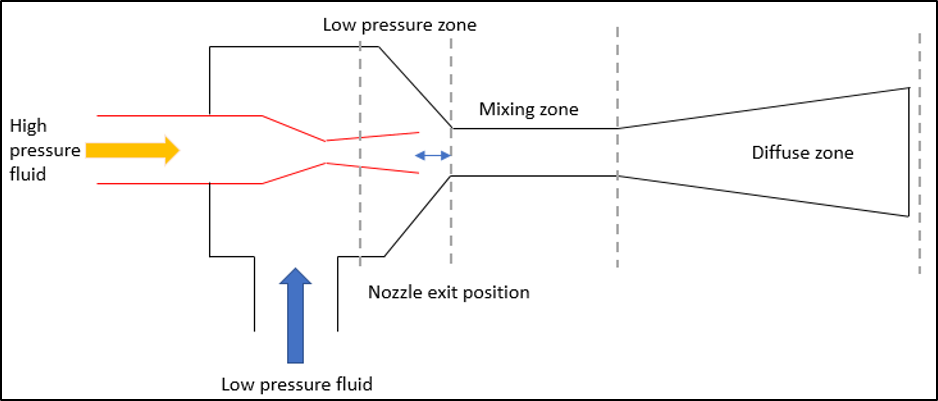# Analysis of Ejector Performance using CFD

#### Introduction

Ejectors are jet devices that uses a high-pressure fluid to entrain a lower pressure fluid, which will then be mixed and discharged at an intermediate pressure. The device is straightforward, require little to no maintenance and it does not have any moving parts making it more cost effective and reliable as compare to the widespread compressors and pumps. The device also allow various fluids to be used which further increases the adaptability of these devices.Figure 1: Typical geometry of the ejector.

The simplest ejector consists of a converging-diverging nozzle, a high-pressure inlet, suction inlet, a mixing chamber and a narrow-to-wide diffuser as shown in Figure 1. A primary fluid of high pressure enters a nozzle where the pressure is converted into high velocity. This creates a vacuum at the nozzle outlet, thus allowing the secondary suction fluid to be entrained and mixed with the primary fluid deriving from indifferent pressure. Following mixed fluid enters a diffuser where the pressure is recovered from the high kinetic energy. The pressure of the fluid at the outlet may be up to 10 times higher than the suction pressure – depending on the geometry, working fluid and other conditions of the ejector.

#### Problem Statement

Ejectors are typically being applied in place of a compressor in refrigeration systems. It is also viable in the oil and gas industry as it can be used in a flare gas recovery system, and to re-start dead wells and boost production. Although these devices are versatile, they are not applied as extensively due lack of knowledge as there are difficulties in physical experimentation and inadequacies in mathematical modelling. This issue may be alleviated especially through the use of computational fluid dynamics (CFD) which enables ejectors to be modeled in 3-D and optimized to utilize its full potential in all applications.

The performance of every ejector is unique and must be optimized through a case-by-case basis where the operating conditions, working fluid and ejector geometry are different. Typically, the performance is measured by the compression ratio and the entrainment ratio which is the ability of the high-pressure fluid to entrain the low-pressure fluid. This depends on many factors, for example, mixing chamber diameter, diffuser chamber diverging angle and distance from HP fluid nozzle exit to the mixing chamber will also affect the efficiency of the ejector.

CFD can be used to investigate the effect of the nozzle exit position (NXP) and pressure ratio of an ejector thus determine the performance of the ejector. Further study can be done on the geometry (i.e. the diameter of the mixing chamber and the diffuser chamber) to obtain an optimized geometry for the specific range of operation.

#### Methodology

The nozzle exit position (NXP) which is the distance between the throat and the mixing chamber of the ejector (as shown in Figure 1) is altered to determine how it accounts for the changes of the entrainment ratio, which is given by-

Entrainmnent Ratio, ER= MS/ MP;

Where the ms is mass flowrate of the suction fluid and mp is the mass flowrate of the high-pressure fluid.

Additionally, the inlet pressure and outlet pressure are varied to determine the highest entrainment ratio.

#### CFD Analysis & Result

From the CFD simulation result which is tabulated in Table 1, it is observed that the nozzle position affects the entrainment ratio, whereby a shorter distance from the mixing chamber proves to have higher entrainment ratio.

When the inlet pressure is varied (as shown in Figure 2), the entrainment ratio increases until a maximum but slowly deteriorates when the inlet pressure is further increased. The optimum value is obtained when the inlet pressure is between 4 and 4.25 MPa which provides the highest entrainment ratio.

 HP inlet (MPa) Suction inlet (MPa) Discharge pressure (MPa) NXP (mm) Entrainment Ratio 3.6 0.4 1 2 0.193 5 0.168 10 0.057

Table 1: Varying the Nozzle Exit Position (NXP)